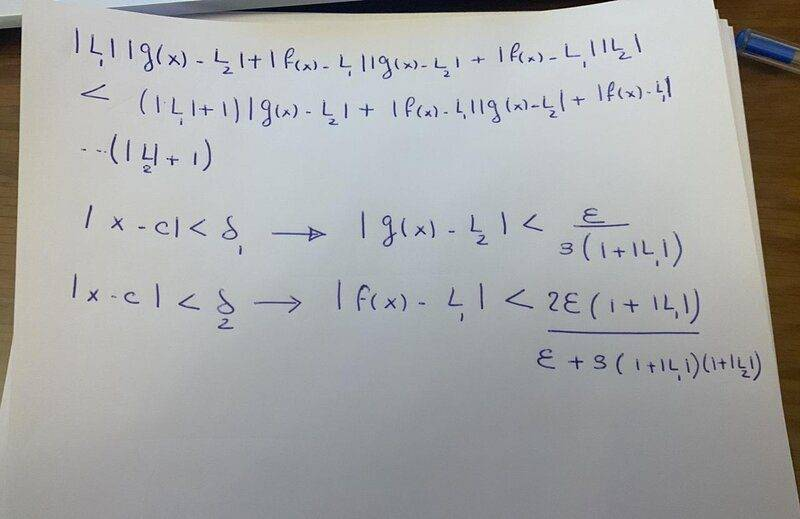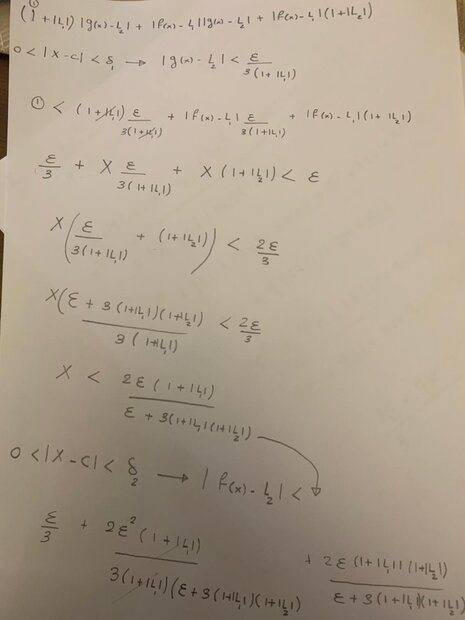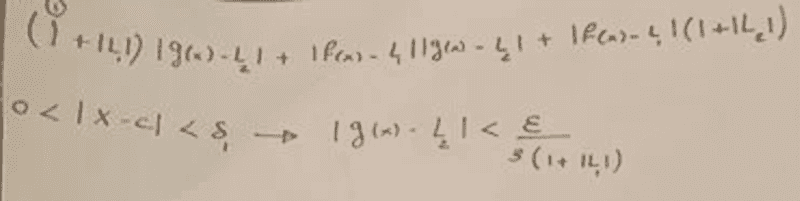# Limit of a product of two functions

In summary, given the conditions of 0< |x-c| <δ -> |f(x)-L1|<ϵ1 and |g(x)-L2|<ϵ2, it can be shown that the product of the absolute values of f(x) and g(x) is equal to the absolute value of their product. By definition, h(x)=f(x)g(x) and it can be shown that the limit of h(x) is equal to the product of the limits of f(x) and g(x). This can be proven by using basic properties of limits and the fact that if the limit of a function is zero, then the function itself is also zero in the neighbourhood of x=c. Therefore,f

Homework Statement
Suppose Lim x->c f(x)=L1 and Lim x->c g(x)=L2. Define h by h(x)=f(x)g(x). Prove that Lim x->c h(x)=L1L2
Relevant Equations
0< |x-c| <δ -> |f(x)-L1|<ϵ1 and |g(x)-L2|<ϵ2
When I look at a range of inputs around x=c and consider the corresponding range of outputs
If 0< |x-c| <δ -> |f(x)-L1|<ϵ1 and |g(x)-L2|<ϵ2 as we shrink the range of inputs the corresponding outputs f(x) and g(x) narrow on L1 and L2 respectively.
|f(x)-L1||g(x)-L2|<ϵ2ϵ1
The product of the absolute values is equal to the absolute value of the product.
|f(x)g(x)-f(x)L2-g(x)L1+L1L2|<ϵ2ϵ1
By definition h(x)=f(x)g(x)
|h(x)-f(x)L2-g(x)L1+L1L2|
|(h(x)-L1L2)-f(x)L2-g(x)L1+2L1L2|
|(h(x)-L1L2)+L2(L1-f(x))+L1(L2-g(x))|<ϵ2ϵ1
The absolute value of a sum is less than or equal to the sum of their absolute values.
|(h(x)-L1L2)+L2(L1-f(x))+L1(L2-g(x))|<|(h(x)-L1L2)|+|L2||(L1-f(x))|+|L1||(L2-g(x))||<|(h(x)-L1L2)|+|L2|ϵ1+|L1|ϵ2
For |(h(x)-L1L2)|+|L2|ϵ1+|L1|ϵ2 to be less than ϵ2ϵ1
|(h(x)-L1L2)|<= ϵ2ϵ1-(|L2|ϵ1+|L1|ϵ2)
(|L1|/ϵ1)+(|L2|/ϵ2)<1
Does this imply that the epsilons must be greater than the limits to guarantee that the sum of the quotients be less than 1

Last edited:
Does this imply that the epsilons must be greater than the limits to guarantee that the sum of the quotients be less than 1
No you don't need that assumption.
Instead of the approach above, focus on the amount you need to show shrinks to zero, which is:
\begin{align*}
|f(x)g(x)-L1\,L2|
&=
|f(x)g(x) - f(x)L2 + f(x)L2 - L1\,L2|
\\&\leq
|f(x)|\,|g(x)- L2| + |f(x)-L1|\,|L2|
\\&=
|L1+f(x)-L1|\,|g(x)- L2| + |f(x)-L1|\,|L2|
\\&<=
(|L1|+|f(x)-L1|)|g(x)- L2| + |f(x)-L1|\,|L2|
\\&<=
|L1|\,|g(x)- L2|+|f(x)-L1|\,|g(x)- L2| + |f(x)-L1|\,|L2|
\end{align*}
From which you can easily deduce the required inequalities by choosing ##\delta_1,\delta_2## appropriately.

•PeroK
Here's an alternative idea:

1a) Prove that if ##\lim_{x \to c} f(x) = 0## and ##\lim_{x \to c} g(x) = 0## then ##\lim_{x \to c}f(x)g(x) = 0##.

1b) Prove or use the fact that if ##\lim_{x \to c} f(x) = L##, then ##\lim_{x \to c} af(x) = aL## (for ##a \in \mathbb R##).

1c) Prove or use the fact that if ##\lim_{x \to c} f(x) = L_1## and ##\lim_{x \to c} g(x) = L_2##, then ##\lim_{x \to c} (f(x) +g(x)) = L_1 + L_2##

2) If ##\lim_{x \to c} f(x) = L_1## and ##\lim_{x \to c} g(x) = L_2##, then consider $$h(x) = (f(x) - L_1)(g(x) - L_2)$$ so that$$f(x)g(x) = h(x) + L_2f(x) + L_1g(x) -L_1L_2$$ And note that the RHS has limit ##L_1L_2##.

I think that provides a proof using only basic already-proved properties of limits, plus 1a).

No you don't need that assumption.
Instead of the approach above, focus on the amount you need to show shrinks to zero, which is:
\begin{align*}
|f(x)g(x)-L1\,L2|
&=
|f(x)g(x) - f(x)L2 + f(x)L2 - L1\,L2|
\\&\leq
|f(x)|\,|g(x)- L2| + |f(x)-L1|\,|L2|
\\&=
|L1+f(x)-L1|\,|g(x)- L2| + |f(x)-L1|\,|L2|
\\&<=
(|L1|+|f(x)-L1|)|g(x)- L2| + |f(x)-L1|\,|L2|
\\&<=
|L1|\,|g(x)- L2|+|f(x)-L1|\,|g(x)- L2| + |f(x)-L1|\,|L2|
\end{align*}
From which you can easily deduce the required inequalities by choosing ##\delta_1,\delta_2## appropriately.
In second expression |g(x)- L2| and |f(x)- L1| can be made as small as wish and f(x) and L2 are bounded in a neighbourhood of x=c (because L2 is constant and f(x) because its limit exists). For a given ϵ it is sufficient to make the expression less than ϵ if each of the terms can be made less than ϵ/2
For a range of inputs δ1 we can choose a number K greater than the corresponding outputs to serve as an upper bound for f(x) . Namely, we can find a δ1 such that 0<|x-c|<δ1-->|f(x)|<=K. K is not allowed to equal zero (no real number is less than zero in magnitude). If K=0 then f(x)=0 in the neighbourhood of x=c. This is reduced to a case of taking the limit of the product of a zero function f(x) and g(x). If K=0, then we can find another number δ2 such that 0<|x-c|<δ2--> |g(x)-L2|<ϵ/2K.
In a similar way since Lim x->f(x)=L1 we can find a number δ3 such that 0<|x-c|<δ3--> |f(x)-L1|<ϵ/2|L2|
If L2=0 by the same reasoning above (K=0) Lim x-> h(x)=0
If L2≠0 we choose the minimum of δ1,δ2, and δ3 for the result to hold.

In second expression |g(x)- L2| and |f(x)- L1| can be made as small as wish and f(x) and L2 are bounded in a neighbourhood of x=c (because L2 is constant and f(x) because its limit exists). For a given ϵ it is sufficient to make the expression less than ϵ if each of the terms can be made less than ϵ/2
For a range of inputs δ1 we can choose a number K greater than the corresponding outputs to serve as an upper bound for f(x) . Namely, we can find a δ1 such that 0<|x-c|<δ1-->|f(x)|<=K. K is not allowed to equal zero (no real number is less than zero in magnitude). If K=0 then f(x)=0 in the neighbourhood of x=c. This is reduced to a case of taking the limit of the product of a zero function f(x) and g(x). If K=0, then we can find another number δ2 such that 0<|x-c|<δ2--> |g(x)-L2|<ϵ/2K.
In a similar way since Lim x->f(x)=L1 we can find a number δ3 such that 0<|x-c|<δ3--> |f(x)-L1|<ϵ/2|L2|
If L2=0 by the same reasoning above (K=0) Lim x-> h(x)=0
If L2≠0 we choose the minimum of δ1,δ2, and δ3 for the result to hold.
That's not too bad, but I think you could avoid the tangle over ##K = 0##. And, you still need to put everything together.

1) It's not a bad idea to deal with the case that ##L_1 = 0## first (that's an easier warm-up proof) and then assume that ##L_1, L_2 \ne 0## in the main proof.

2) Alternatively, replace ##|L_1|## by ##|L_1| + 1## and the same for ##L_2## in Andrew's last inequality. This solves the problem of ##L_1 = 0## etc.

Note that the middle term is a little problematic:$$|f(x)-L1|\,|g(x)- L2|$$An idea to deal with that is:

3) Choose some ##\delta_f## such that ##|f(x) - L1| < 1##, which can simplify the middle term.

Note that all this trickiness is good practice!

|L1||g(x)-L2|+x-c|+|f(x)-L1||g(x)-L2|+|f(x)-L1||L2|<(1+|L1|)|g(x)-L2|+x-c|+|f(x)-L1||g(x)-L2|+|f(x)-L1|(1+|L2|)
We know there exists a δ1 such that when 0<|x-c|<δ1->|g(x)-L2|<(ϵ/3(1+|L1|). In a similar way, there exists a δ2 such that 0<|x-c|<δ2->|f(x)-L1|<2ϵ(1+|L1|)/(ϵ+3(1+|L1|)(1+|L2|))
(1+|L1|)|g(x)-L2|+x-c|+|f(x)-L1||g(x)-L2|+|f(x)-L1|(1+|L2|)<ϵ

|L1||g(x)-L2|+x-c|+|f(x)-L1||g(x)-L2|+|f(x)-L1||L2|<(1+|L1|)|g(x)-L2|+x-c|+|f(x)-L1||g(x)-L2|+|f(x)-L1|(1+|L2|)
We know there exists a δ1 such that when 0<|x-c|<δ1->|g(x)-L2|<(ϵ/3(1+|L1|). In a similar way, there exists a δ2 such that 0<|x-c|<δ2->|f(x)-L1|<2ϵ(1+|L1|)/(ϵ+3(1+|L1|)(1+|L2|))
(1+|L1|)|g(x)-L2|+x-c|+|f(x)-L1||g(x)-L2|+|f(x)-L1|(1+|L2|)<ϵ
That's hard to read. Can you not try Latex, perhaps using post #2 as a starting point?I'm not sure about that second line. You'd be better just to do the same for ##f(x) - L_1## as you did for ##g(x) - L_2##, and then find a third delta for the middle term.

If you want to know how I obtained the second line, first, I denoted f(x)-L1 by any arbitary letter and treated it as an unknown for which the RHS of the inequality is less than ϵ and made use of the first line.

If you want to know how I obtained the second line, first, I denoted f(x)-L1 by any arbitary letter and treated it as an unknown for which the RHS of the inequality is less than ϵ and made use of the first line.
I'm not convinced. In any case, you have to show that choice of ##\delta## leads to the required inequality. It's not obvious from what you've written.

So for the choice δ1 and by treating f(x)-L1 as an unknown. There are 2 possible scenarios that could happen. That for this range of input within δ1 the corresponding outputs of f(x) when plugged in the inequality will yield a result greater than ϵ or less than ϵ. So I constrained the result to be less than ϵ and found the second line. In doing so is δ2 a sub-interval of δ1? If δ2>δ1, If we use the minimum namely δ1 the expression will be less than ϵ and vice versa.

So for the choice δ1 and by treating f(x)-L1 as an unknown. There are 2 possible scenarios that could happen. That for this range of input within δ1 the corresponding outputs of f(x) when plugged in the inequality will yield a result greater than ϵ or less than ϵ. So I constrained the result to be less than ϵ and found the second line. In doing so is δ2 a sub-interval of δ1? If δ2>δ1, If we use the minimum namely δ1 the expression will be less than ϵ and vice versa.
Let's see the maths! This is too complicated, in any case. You need to avoid this sort of tangle.Sorry, I don't see a proof there. It just ends with some out-of-control algebra.

I suggest that to deal with the middle term, you choose ##\delta_3## s.t ##|g(x) - L_2| < 1##. Then you have three terms, all less than ##\epsilon/3##.

•I'm not sure that you're totally clear on the algebra and/or the overall inequality.

Following the steps of @andrewkirk in Post #2, but only using his grouping without any recourse to absolute values gives the following equality.

##\displaystyle \left( f(x)g(x)-L_1\,L_2 \right) = \left(L_1\right)\,\left(g(x)- L_2\right)+\left(f(x)-L_1\right)\,\left(g(x)- L_2\right) + \left(f(x)-L_1\right)\,\left(L_2 \right) ##

Now taking the absolute value of both sides, followed by using the triangle inequality for the three terms on the right hand side will give the desired result.

Furthermore, if you want to avoid the trick of adding and subtracting terms seemingly chosen at 'random', you might try expanding, the simplifying the following expression. (Perhaps, seemingly chosen at 'random')

##\displaystyle \left( f(x)g(x)-L_1\,L_2 \right) - \left( \left(f(x)-L_1\right)\,\left(g(x)- L_2\right) \right)##

A snip from your Post #14 image:There is no need to add 1 to |L1| and |L2| in that first line.

Just so you add 1 (some a positive number) to |L1| in the denominator of that last expression in the second line. That will take care of a zero limit as @PeroK said.

Then if ##\delta_1 ## is chosen so that ##\displaystyle \ \left| g(x) - L_2 \right| < \frac{\varepsilon}{3\left( 1 + \left| L_1 \right| \right) } ## ,
you will have that
##\displaystyle \left| L_1 \right| \left| g(x) - L_2 \right| < \frac{ \varepsilon \left| L_1 \right| }{3\left( 1 + \left| L_1 \right| \right) } ## which is clearly less that ## \dfrac{\varepsilon}{3 } ## .

Last edited: